# Multi-period Returns

## Compounded Returns

While one-period returns may be normally distributed, this will generally not be the case for the value of a portfolio many periods hence, due to the effects of compounding. If \$1 is invested initially, the value will be (1+r1) at the end of period 1, where r1 is the rate of return in the first period. If money is neither withdrawn from nor added to the account so that (1+r1) is invested at the beginning of period 2, the value at the end of period 2 (v2) will be (1+r1)*(1+r2), where r2 is the rate of return in the second period:

```   v2 = (1+r1)*(1+r2)
```

Equivalently:

```   v2 = 1 + r1 + r2 + r1*r2
```

The final term reflects the effects of compounding. If r1 and r2 are normally distributed, r1*r2 will not be, and hence neither will v2.

Assume that in each period there is a 0.50 probability that \$1 will become (e+sd) and a 0.50 probability that it will become (e-sd). For example, if e = 1.10 and sd=0.15: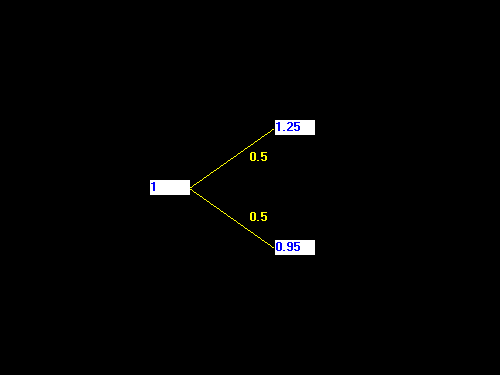Note that this is not a normal distribution, but it is symmetric, with an expected value relative of e and a standard deviation of sd.

With compounding, the ending values after two periods for an initial investment of \$1 would be those shown in the following diagram: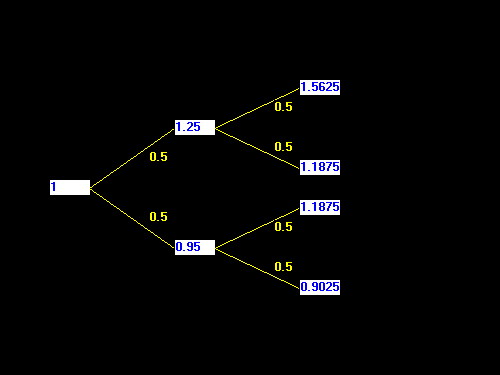A somewhat different representation shows the one-period value relatives at each node in the diagram, rather than the cumulative values: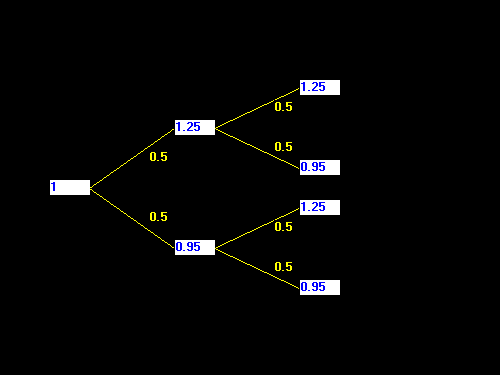In this case the one-period value relative distribution is the same at all points in the diagram: neither the ending values nor the probabilities associated with the branches change through time or depend on prior outcomes. Such returns are said to be independent and identically distributed (iid, for short). Since the distribution of possible one-period returns looks the same in such a situation, no matter what has happened in the past, returns can be said to follow a random walk.

Note that this type of tree "folds back" on itself, so that there are only three distinct outcomes:

```   (e+sd)*(e+sd)       : probability = 0.25
(e+sd)*(e-sd)       : probability = 0.50
(e-sd)*(e-sd)       : probability = 0.25
```

Expanding:

```  e^2 + 2*sd + sd^2  : probability = 0.25
e^2        - sd^2  : probability = 0.50
e^2 - 2*sd + sd^2  : probability = 0.25
```

The expected ending value is found by weighting each outcome by its probability. In this case it will be:

```   ev2 = e^2
```

Perhaps not surprisingly, the two-period expected value is simply the one-period expected value relative squared.

There is more to be said, however. Consider the distribution of the ending values: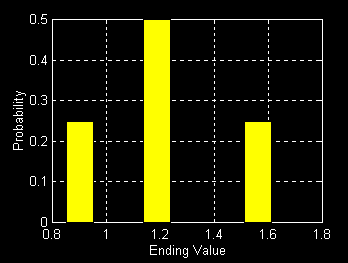Note that the distribution is not symmetric, since the largest value is farther to the right of the most likely value than the smallest value is to the left of the most likely value. The distribution is skewed to the right. Note also that 1.1875 (=0.95*1.25), the most likely outcome, known also as the mode, is smaller than the expected outcome (1.1^2=1.21).

In our two-period case the most likely outcome (e+sd)*(e-sd) is also the median outcome: the probability of a smaller value is equal to the probability of a larger value. It is thus of considerable interest. Its value is:

```  e^2 - sd^2
```

which is equal to the expected one-period value relative squared minus the one-period variance.

It is convenient to translate this ending value into a "what if" value called, in some contexts, the geometric mean -- the return per period which, if obtained with no variance, would have produced the same ending value. Here:

```   (1+g)^2 = e^2 - sd^2
```

or:

```  (1+g)^2 = (1+er)^2 - sd^2
```

where er is the one-period expected return. In this case:

`  (1+g)^2 = (1 + .10)^2 - 0.15^2 = 1.21 - .0225 = 0.1875`

and

```   1 + g = sqrt(1.1875) =  1.0897
```

Thus g = 8.97%, which is less than 10.0%, the one-period expected return.

While this expression is perfectly usable, practitioners often adopt a simpler approximation. Expanding the squared expressions gives:

```  1 + 2*g + g^2 = 1 + 2*er + er^2 - sd^2
```

or:

```  er - g = (g^2 - er^2)/2 + (sd^2)/2
```

Since er and g are generally significantly less than one (e.g. 0.10 and 0.09), both er^2 and g^2 will be even smaller (e.g. 0.0100 and 0.0081). Moreover, half the difference between g^2 and er^2 will be even smaller yet (e.g. -0.00095). Hence it will be approximately true that:

```  er - g = (sd^2)/2
```

or

```  g = er - (sd^2)/2
```

For example, if er = 0.10 and sd = 0.15, then:

```  g = 0.10 - 0.0225/2 = 0.10 - 0.01125 = 0.08875
```

or 8.875%, only slightly different from the more precise estimate of 8.97%.

If the return on a diversified stock market portfolio is assumed to be iid with a standard deviation of 15% per year, the median long-term return (g) will be approximately 1.125% ((0.15^2)/2) below the expected one-period return (e). If the standard deviation of return were 20%, the difference would be 2.0% ((0.20^2)/2). And so on.

The geometric mean return will be less than the expected return (sometimes termed the arithmetic mean), as long as there is some variation in returns. Moreover, the difference between the geometric and arithmetic means will be greater, the greater the amount of such variance.

What about longer periods? Consider the ending value of a portfolio n periods hence, where n is an even number. The most likely and median outcome will have n/2 "up moves" and n/2 "down moves". Hence, the n-period median ending value (evn) will be:

```  evn  = ( (e+sd)^(n/2))*((e-sd)^(n/2) )
= ((e+sd)*(e-sd))^(n/2)
= (e^2 - sd^2)^(n/2)
```

The geometric mean will be the value that satisfies:

```   (1+g)^n = (e^2 - sd^2)^(n/2)
```

or:

```  ((1+g)^2)^(n/2) = (e^2 - sd^2)^(n/2)
```

or:

```  (1+g)^2 = e^2  - sd^2
```

which is precisely the relationship found earlier.

## Lognormal Distributions

It is common in asset allocation studies to assume that returns are independent and identically distributed. This has important implications for the distribution of long-term returns.

Let v1,v2,...,vn be the value relatives for a portfolio in periods 1,2,..,n, respectively. Assuming an initial investment of \$1 with periodic compounding and no withdrawals or additional investments, the ending value in period n will be:

```  evn = v1*v2*...*vn
```

Now, take the logarithm of each side:

```  ln(evn) = ln(v1*v2*...*vn)
```

or:

```   ln(evn) = ln(v1) + ln(v2) + ... + ln(vn)
```

Ex ante, each of the variables on the right-hand side is unknown. Each will be drawn from a distribution (that of ln(v)) and each draw will, by assumption, be independent of every other draw.

Recall the central limit theorem, which holds that the sum of a set of independent random variables will have a distribution that will be closer and closer to normal, the greater the number of variables in the sum. For a sufficiently large value of n, ln(evn) will be normally distributed, or nearly so.

We say that variable x has a lognormal distribution if the distribution of ln(x) is normal. Thus long-term compounded values tend to be lognormally distributed if returns are independent. Note that this result follows, no matter what the distributions of one-period returns may be, as long as the returns are independent.

The figures below show distributions of ln(evn) and evn when evn is lognormally distributed.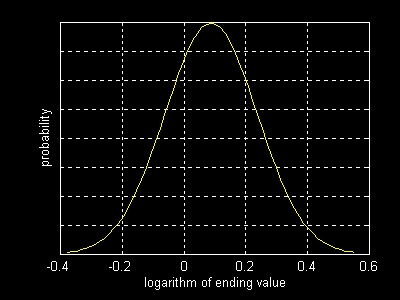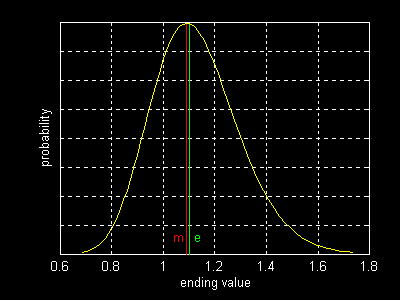Note that the distribution of evn is skewed to the right, due to the relationship between evn and ln(evn) and the symmetry of the distribution of the latter. Note also that m -- the modal and median value of evn will be less than e, the expected value.

To fix ideas, consider the two-period example discussed earlier. The one-period value relatives are:

```   e+sd  with probability = 0.50
e-sd  with probability = 0.50
```

In logarithmic terms:

```  ln(e+sd) with probability = 0.50
ln(e-sd) with probability = 0.50
```

The expected logarithm is thus:

```  0.50*ln(e+sd) + 0.50*ln(e-sd)
= 0.50*(ln(e+sd)+ln(e-sd)
= 0.50*ln((e+sd)*(e-sd))
= 0.50*ln(e^2 - sd^2)
```

Let ln(1+g) represent this mean (for reasons that will become clear shortly). Then:

```  ln(1+g) = 0.50*ln(e^2 - sd^2)
```

and:

```  (1+g)^2 = e^2 - sd^2
```

which is the formula obtained earlier for the geometric mean.

We know that ln(1+g) is the mean of the distribution of ln(ev1). It follows that n*ln(1+g) is the mean of the distribution of ln(evn). But since the modal (median) ending value will equal the exponential of the mean value of the logarithm:

```  median(evn) = (1+g)^n
```

Thus the median outcome will equal the value obtained by compounding each period at the geometric mean rate of return. There is a 50% chance that the actual value will exceed this amount and a 50% chance that it will fall below it.

In some cases it is necessary to determine the moments of the distribution of the logarithm of a lognormally-distributed value from those of the value itself or vice-versa. The formulas for doing so are slightly complicated but easily computed. Assume that log(y) is normally distributed with mean el and standard deviation sl. Then the mean (e), variance (v) and standard deviation (s) of y will equal:

e = exp ( el + ( ( sl^2 ) / 2 ));
v = exp ( 2*el + ( sl^2 ) ) * ( exp (sl^2) - 1);
s = sqrt ( v );

where:

exp ( z ) = the exponential of z (that is, e raised to the z'th power)

If the mean and variance of y are known, the moments for the distribution of log(y) can be found by sequentially evaulating the equations below:

b = sqrt ( log ( ( v / (e^2) ) + 1) );
a = 0.5 * log ( (e^2) / exp(b^2) );

where:

log ( z ) = the natural logarithm of z

## Discounting Projected Values

In corporate finance and investment practice it is common to project a set of cash flows, then discount them using an appropriate cost of capital or discount rate. If the resulting value is less than the cost of the investment, it is rejected. If the value exceeds the cost, the investment is accepted. Key to the validity of such a procedure is the choice of an appropriate cost of capital or discount rate.

We will not attempt a complete discussion of this topic, but it is useful to analyze the arguments for using a geometric mean vis-a-vis an arithmetic mean for such purposes.

Consider our example in which a standard market investment produces a return of (e+sd) with probability 0.50 and a return of (e-sd) with probability 0.50 in each period. The expected cost of capital for such an investment is e, while the geometric mean is given by:

```  (1+g)^2 = e^2 - sd^2
```

Now consider a project that is expected to make a payment two periods hence of:

```  (e+sd)*(e+sd)  with probability 0.25
(e+sd)*(e-sd)  with probability 0.50
(e-sd)*(e-sd)  with probability 0.25
```

We know that such a project is worth \$1 since its payments can be replicated in the market for this amount.

In practice those charged with assessing the project will be asked to produce a single set of cash flows over time (in this case, one number for the ending cash flow). A discount rate will then be used to compute the present value.

If the project's cash flow is implicitly or explicitly estimated by taking all possibilities into account as well as the associated probabilities, the result will be equivalent to an expected value -- in this case, e^2. Clearly, such an estimate should be discounted using the expected return (arithmetic mean). Here:

```  (e^2)/(e^2) = 1
```

which is the correct present value. This is the method advocated by many who have addressed the issue. However the argument for using the expected return as a discount rate assumes that that the projection process takes into account all possible future cash flows and the accompanying probabilities. In many cases a much simpler approach is utilized. Imagine a situation in which only the most likely (or "50/50") outcome was considered. In our example, the projected cash flow would then be:

```  (e+sd)*(e-sd) = (1+g)^2
```

If this were discounted using the expected cost of capital, the resultant value would be less than \$1 -- clearly a wrong answer. The correct value would be obtained by discounting with the geometric mean:

```  ((1+g)^2)/((1+g)^2) = 1
```

In practice cash flows are projected for many different periods. Moreover, the assumptions utilized to make such projections are often highly implicit. Those making projections may even adjust their estimates to assure a particular outcome if the "hurdle rate" (cost of capital) is known beforehand. Thus the nature of the overall process must be known before a "theoretically correct" procedure can be determined. In some cases an expected return may be appropriate discount rate, but in many instances a geometric mean (median return) may provide more correct results.